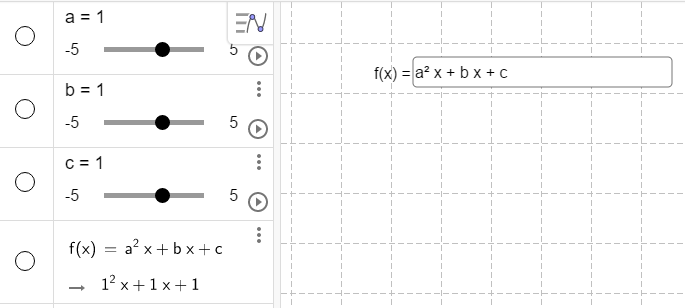# Show • between letters in the input box

Aritmometer shared this problem 2 years ago
Not a Problem

Show • between letters in the input box.

So when for example when the formula b^2 - 4 • a • c is shown in the input box, the students just have to substitute the letters with numbers without the need to also write • between the numbers.

If the students don’t do this, the program will stall.

As an alternative, you could make a error-message saying that there have to be placed some operation signs between the numbers so that the program won’t stall and the students become aware of the cause and what to change and remember next time.

But of cause it would be easier for the students with the first solution.

A third alternative would be to make it possible to convert a string to a function without the expression is reduced. That would also make it possible to use input boxes for this purpose without the students have to delete the content of the input box before writing a new input and without having a content that can misguide the students.1

You can make seperate inputboxes for a, b and c and then show the expression as a dynamic text that isn't reduced.

chris1

Yes, but the idea is to make assignments where the students is going to use a formula and get GeoGebra to both check whether they have inserted the numbers in the formula correctly, reduced the formula correctly (without a calculator) and is able to get the right result.

In this way it is much easier for the students to find an error in their calculation and correct it without the need of a teacher to do the same. And in this way the teacher get more time to help students with other problems.1

Yes, but the idea is to make assignments where the students is going to use a formula and get GeoGebra to both check whether they have inserted the numbers in the formula correctly, reduced the formula correctly (without a calculator) and is able to get the right result.

In this way it is much easier for the students to find an error in their calculation and correct it without the need of a teacher to do the same. Thereby the students will learn to use the formula much faster and the teacher get more time to help students with other problems.1

If you define a function f as f(x)= ax² + b x + c sliders for a, b and c will be created automatically in GG6 but that's no problem. If you now make an inputbox for f and mark it as symbolic, it will show the formula. Now students can replace the letters a, b or c by whatever number, that's no problem. So I guess that's what you wanted. You can do it also in GG5.

chris1

Sorry, I think you missed the point of my question.

The idea is to have a formula like for example “b^2 - 4 * a *c” written in a input box where the students just have to substitute the letters with numbers.

The reason for this is to make it easy for the student to insert integers in the formula and to get GeoGebra to check if they have inserted the integers correctly in the formula.

At this moment when you create an input box and write “b^2 - 4 * a *c” in it, then “b^2 - 4ac” will be written in it. And if the students just substitute the letters with integers without writing “*” in between a and c, then GeoGebra will stall.

Therefore I suggest to make it possible to get “•” written between letters in an input box.

This can for example be done by adding an option to the properties of an input box: “Show • between letters”.1

Sorry, I think you missed the point of my question.

The idea is to have a formula like for example “b^2 - 4 * a *c” written in a input box where the students just have to substitute the letters with numbers.

The reason for this is to make it easy for the student to insert integers in the formula and to get GeoGebra to check if they have inserted the integers correctly in the formula.

At this moment when you create an input box and write “b^2 - 4 * a *c” in it, then “b^2 - 4ac” will be written in it. And if the students just substitute the letters with integers without writing “*” in between a and c, then GeoGebra will stall.

Therefore I suggest to make it possible to get “•” written between letters in an input box.

This can for example be done by adding an option to the properties of an input box: “Show • between letters”.1

Sorry, we won't be adding this